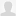# gnarl,TopCoder

## 2010-02-22

### Topcoder SRM 461 Div2

23:40

#### 250 TrappingRabbit

##### 問題

There is a grass field that is represented by a 1000 by 1000 grid. Initially, a rabbit is present on the square located at coordinates (1,1) (1-based). The rabbit can move to a horizontally or vertically adjacent square in one second and can only move that way.

You are going to trap the rabbit. To do so, you have set some traps. The i-th trap is located on a square given by trapX[i] and trapY[i] as its X and Y coordinates (1-based) respectively.

Return the minimum number of seconds so that after this time has passed there is a non-zero chance that the rabbit has fallen into one of your traps (the rabbit falls into one of your traps if it is in the same square as one of your traps).

http://www.topcoder.com/stat?c=problem_statement&pm=10749&rd=14181&rm=303574&cr=22717385

1000x1000の格子で区切られたフィールドがある。座標(1,1)(1-origin)にウサギがいる。ウサギは1秒ごとに上下左右いずれかへ1ブロック移動できる。

##### 回答

http://www.topcoder.com/stat?c=problem_solution&rm=303574&rd=14181&pm=10749&cr=22717385

```public class TrappingRabbit {

public int findMinimumTime(int[] trapX, int[] trapY) {
final int traps = trapX.length;
int min = Integer.MAX_VALUE;
for (int i = 0; i < traps; i++) {
min = Math.min(trapX[i] + trapY[i] - 2, min);
}

return min;
}

}
```

ウサギからすべての罠までのマンハッタン距離を求め、一番近い罠までの移動時間を求めればいい。

passed system test.

#### 500 ColoringRectangle

##### 問題

You are given a white rectangle of size width by height. A green horizontal line (parallel to the width of the rectangle) is drawn through the middle of the rectangle so that it divides the rectangle into two congruent rectangles. This line extends infinitely out of the rectangle. You are asked to place red and blue disks (a disk is a circle and its interior) on the rectangle so that the entire rectangle is covered. The center of every disk must be placed on the green line, not necessarily within the rectangle bounds. Disks are placed sequentially from left to right, i.e., the center of each next placed disk must lie strictly to the right of the center of the last previously placed disk. Each disk is placed on top of all previously placed disks, i.e., when a disk is placed it covers any parts of previously placed disks that overlap. To challenge yourself, you have decided to only allow disk placements that satisfy the following additional constraint.

Every point covered by a newly placed disk must either

not be covered by any previous disk or

if covered by some previous disk then the topmost previous disk covering this point must be a different color than the newly placed disk.

You are given int red and int blue. The number of elements in red and blue corresponds to the number of red and blue disks you have, respectively. Each element of red or blue is the diameter of a red or blue disk, respectively. Note that each disk can only be used at most once. Find the smallest number of disks that can be placed as described above such that every point in the rectangle is covered by at least one disk. Return -1 if this is not possible.

http://www.topcoder.com/stat?c=problem_statement&pm=10731&rd=14181&rm=303574&cr=22717385

• 円盤の中心は、長方形を水平に二等分する線と重ならねばならない(ただし円の中心が長方形の内側にくる必要はない)
• 円盤は左から右へ置かれる。つまりn番目におかれた円盤の中心点はn-1番目の円盤の中心点より右
• 新しく置かれた円盤は、前の円盤の上に置かれる
• 新しく置かれた円盤に覆われる領域は、以下のいずれかを満たすこと
• 他の円盤に覆われていない
• もしくは
• 置こうとしている円盤と違う色で覆われている

##### 回答
• 大きい円盤から順に置く
• 赤青交互に置く
• 直径が長方形の高さより大きい円盤のみを使う

という操作をすれば制約を満たせる。

(僕の説明はわかりづらいですね……)

http://www.topcoder.com/stat?c=problem_solution&rm=303574&rd=14181&pm=10731&cr=22717385

```public class ColoringRectangle {

public int chooseDisks(int width, int height, int[] red, int[] blue) {
Arrays.sort(red);
Arrays.sort(blue);

final int r = cover(width, height, red, blue);
final int b = cover(width, height, blue, red);
final int result = (r == -1) ? b : b == -1 ? r : Math.min(r, b);
System.out.println("result: " + result);
return result;
}

private int cover(int width, int height, int[] first, int[] second) {
System.out.println("=====================");
System.out.println("width: " + width);
int n = 0;
double w = width;
for (int i = 0;; i++) {
if (first.length <= i)
break;
final int fd = fromLast(first, i);
if (fd < height)
return -1;
w -= l(height, fd);
n++;
System.out.println("rest: " + w + " " + n);
if (w < 0)
return n;
if (second.length <= i)
break;
final int sd = fromLast(second, i);
if (sd < height)
return -1;
w -= l(height, sd);
System.out.println("rest: " + w + " " + n);
n++;
if (w < 0)
return n;
}
System.out.println("fail.");
return -1;
}

private int fromLast(int[] array, int i) {
return array[array.length - 1 - i];
}

private double l(double h, double d) {
final double result = d * Math.cos(Math.asin(h / d));
System.out.println("h,d,result=" + h + ", " + d + ", " + result);
return result;
}

}
```

どうにかpassed system test.トラックバック - http://topcoder.g.hatena.ne.jp/gnarl/20100222